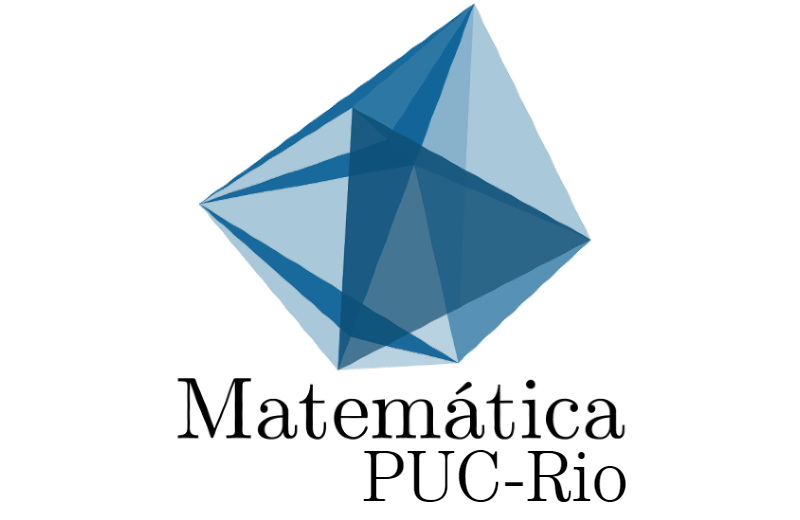Colóquio - Calculus and flows on logarithmic latticesCalculus and flows on logarithmic lattices
Expositor: Alexei A. Mailybaev
Instituição: IMPA
Data e Horário: 12/11/2019 | 17:00 hs

Resumo: Logarithmic lattices are defined as sets of nodes, whose coordinates in each direction are geometric progressions. We show how the usual algebraic operations and calculus can be introduced on such lattices, by mimicking properties of the common Euclidean space. It turns out that such constructions are possible only for special values of the lattice parameter, including for some celebrated algebraic constants: the golden ratio and plastic constant. Then, we demonstrate that some PDEs in Mathematical Physics can be formulated on logarithmic lattices, preserving most of their symmetries and invariants. In particular, the study of a finite-time singularity (blowup) on such lattices gives a new insight to the respective long-standing open problems in Fluid Dynamics. This is a joint work with Ciro Campolina.